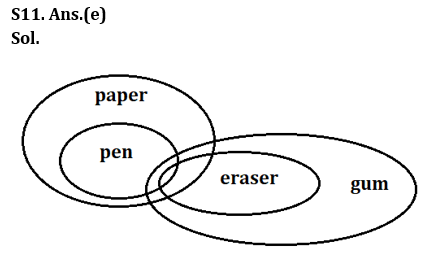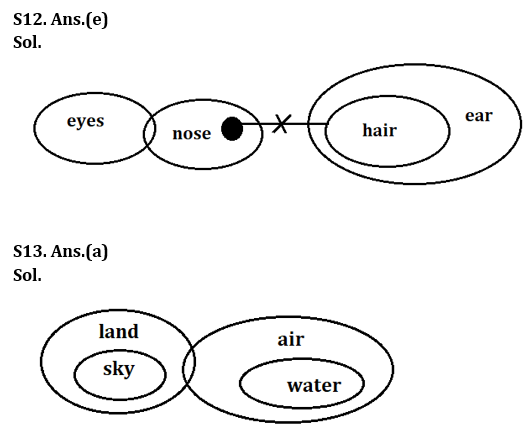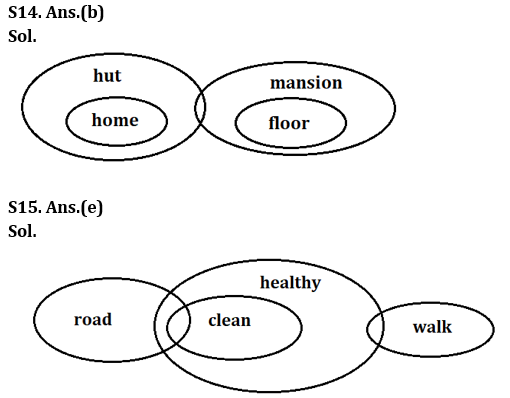Latest Banking jobs   »

# Reasoning Ability Quiz For Bank Foundation 2023-9th March

Direction (1-5): Study the following information and answer the given question below:

9 7 2 9 9 4 8 7 7 2 7 5 9 4 8 2 9 4 6 5 7 1 4 3 2 4 1 6 6 3 9 8 2 2

Q1. How many such odd number are there in the above arrangement, each of which is immediately preceded by an even number and immediately followed by an odd number?
(a) None
(b) One
(c) Two
(d) Three
(e) More than three

Q2. How many such even numbers are there in the above arrangement each of which is immediately preceded by a prime number?
(a) None
(b) One
(c) Two
(d) Three
(e) More than three

Q3. Which of the following is the fifth to the right of the eight from the left end of the above arrangement?
(a) 8
(b) 9
(c) 4
(d) 5
(e) None of these

Q4. Which of the following is the fourth to the right of the twelfth from the right end of the above arrangement?
(a) 1
(b) 6
(c) 9
(d) 4
(e) 2

Q5. If all the prime numbers in the above arrangement are dropped, which of the following will be the sixth from the left end?
(a) 8
(b) 9
(c) 4
(d) 6
(e) None of these

Directions (6-10): These questions are based on the following five numbers:

245 674 978 887 635

Q6. If we arranged all numbers in ascending order from left end then, the position of how many numbers will remain unchanged?
(a) One
(b) None
(c) Three
(d) Two
(e) More than three

Q7. If we interchanged 1st and 3rd digit of each number within the number then, how many numbers thus formed are even numbers?
(a) None
(b) Three
(c) Two
(d) One
(e) More than three

Q8. If we interchanged 1st and 2nd digit of each number within the number then, which of the following number thus formed is 3rd smallest number?
(a) 245
(b) 674
(c) 978
(d) 887
(e) 635

Q9. If we arranged all digit in all numbers in descending order then, which of the following number thus formed is the smallest number?
(a) 245
(b) 674
(c) 978
(d) 887
(e) 635

Q10. What is the sum of 3rd digit of 2nd number from left end and 2nd digit of 3rd number from right end?
(a) 10
(b) 8
(c) 9
(d) 11
(e) None of these

Direction (11-15): In each of the questions below some statements are given followed by two conclusions. You have to take the given statements to be true even if they seem to be at variance with commonly known facts. Read all the conclusions and then decide which of the given conclusions logically follows from the given statements disregarding commonly known facts.
(a) If only conclusion I follows.
(b) If only conclusion II follows.
(c) If either conclusion I or II follows.
(d) If neither conclusion I nor II follows.
(e) If both conclusions I and II follow.

Q11. Statements: All pen is paper.
Some pen is eraser.
All eraser is gum
Conclusions: I. Some gum is paper.
II. Some paper is eraser.

Q12. Statements: Some eyes is nose.
Some nose is not hair.
All hair is ear
Conclusions: I. Some nose can be ear.
II. Some eyes can be hair.

Q13. Statements: Only a few land is air.
Only air is water.
All sky is land.
Conclusions: I. Some air can be sky.
II. Some land can be water.

Q14. Statements: Only hut is home.
Some hut is mansion.
Only mansion is floor.
Conclusions: I. Some home can be floor.
II No floor is hut.

Q15. Statements: Only a few road is clean.
All clean is healthy.
Some healthy is walk.
Conclusions: I. Some road is not clean.
II All walk can be healthy.

Solutions

Solutions (1-5):
S1. Ans. (e)
S2. Ans. (e)
S3. Ans. (b)
Sol. fifth to the right of the eight from the left end of the above arrangement= 5+8=13th from left end= 9
S4. Ans. (a)
Sol. 4th to the right of the 12th from right means = 12-4= 8th from the right, i.e., 1.
S5. Ans. (b)
Sol. 9

Solution (6-10):
S6. Ans. (d)
S7. Ans. (e)
S8. Ans. (b)
S9. Ans. (a)
S10. Ans. (d)## FAQs

### What is the selection process of the Bank Clerk?

The selection process of the Bank Clerk is Prelims & Mains.

#### Congratulations!Union Budget 2023-24: Free PDF